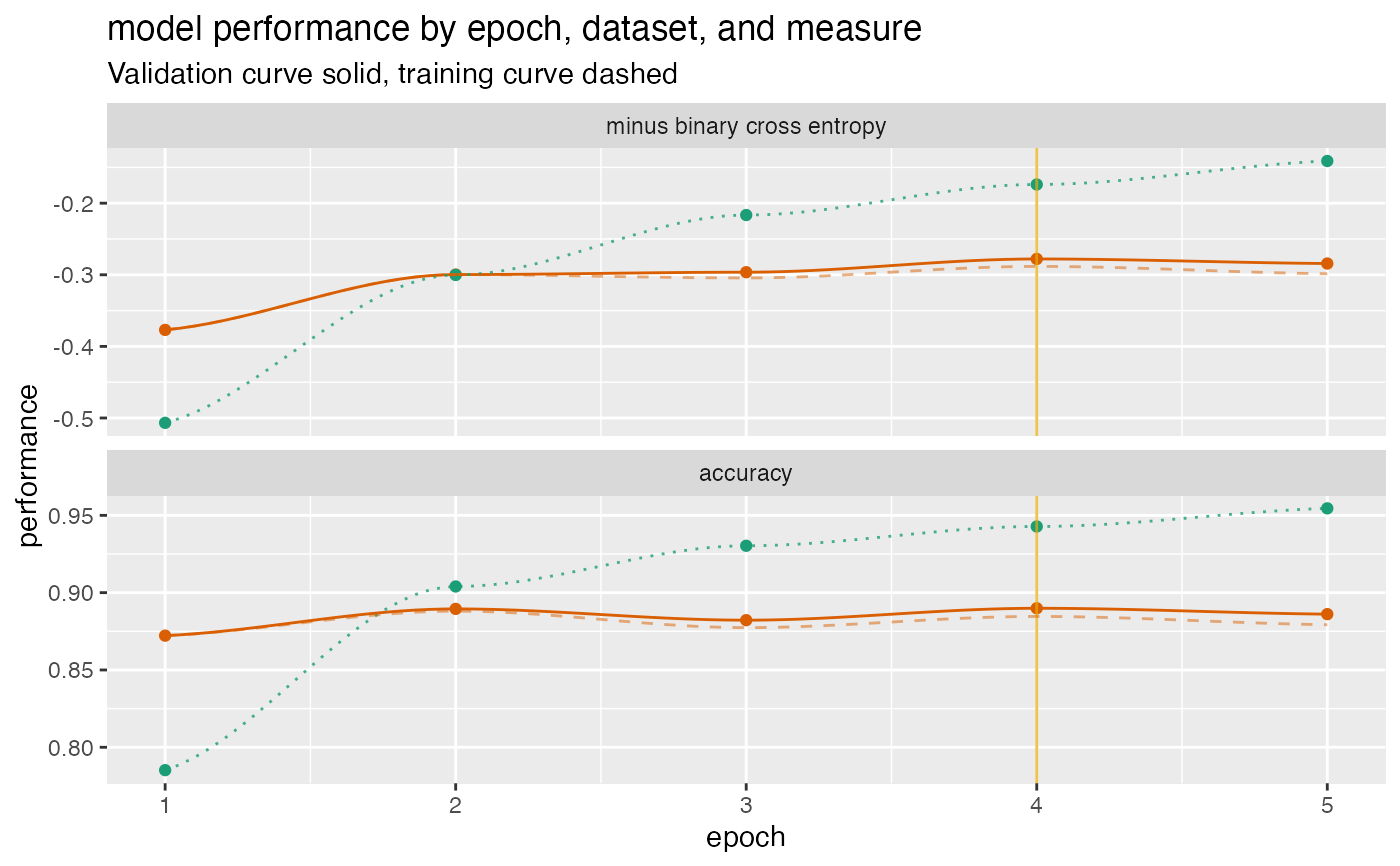Plot a history of model fit performance over the a trajectory of times.

plot_fit_trajectory(
d,
column_description,
title,
...,
epoch_name = "epoch",
needs_flip = c(),
pick_metric = NULL,
discount_rate = NULL,
draw_ribbon = FALSE,
draw_segments = FALSE,
val_color = "#d95f02",
train_color = "#1b9e77",
pick_color = "#e6ab02"
)

## Arguments

d data frame to get values from. description of column measures (data.frame with columns measure, validation, and training). character title for plot. force later arguments to be bound by name name for epoch or trajectory column. character array of measures that need to be flipped. character metric to maximize. numeric what fraction of over-fit to subtract from validation performance. present the difference in training and validation performance as a ribbon rather than two curves? (default FALSE) logical if TRUE draw over-fit/under-fit segments. color for validation performance curve color for training performance curve color for indicating optimal stopping point

ggplot2 plot

## Details

This visualization can be applied to any staged machine learning algorithm. For example one could plot the performance of a gradient boosting machine as a function of the number of trees added. The fit history data should be in the form given in the example below.

The example below gives a fit plot for a history report from Keras R package. Please see https://win-vector.com/2017/12/23/plotting-deep-learning-model-performance-trajectories/ for some examples and details.

## Examples


d <- data.frame(
epoch    = c(1,         2,         3,         4,         5),
val_loss = c(0.3769818, 0.2996994, 0.2963943, 0.2779052, 0.2842501),
val_acc  = c(0.8722000, 0.8895000, 0.8822000, 0.8899000, 0.8861000),
loss     = c(0.5067290, 0.3002033, 0.2165675, 0.1738829, 0.1410933),
acc      = c(0.7852000, 0.9040000, 0.9303333, 0.9428000, 0.9545333) )

cT <- data.frame(
measure =    c("minus binary cross entropy", "accuracy"),
training =   c("loss",                       "acc"),
validation = c("val_loss",                   "val_acc"),
stringsAsFactors = FALSE)

plt <- plot_fit_trajectory(
d,
column_description = cT,
needs_flip = "minus binary cross entropy",
title = "model performance by epoch, dataset, and measure",
epoch_name = "epoch",
pick_metric = "minus binary cross entropy",
discount_rate = 0.1)

suppressWarnings(print(plt)) # too few points for loess
#> geom_smooth() using formula 'y ~ x'#> geom_smooth() using formula 'y ~ x'#> geom_smooth() using formula 'y ~ x'